#### Forward projections under a fixed plan

Forward projections can now be defined under the constraint that a particular plan is executed. The specific expression of actions is replaced by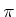. Each time an action is needed from a state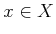, it is obtained as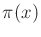. In this formulation, a different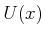may be used for each, assuming thatis correctly defined to use whatever actions are actually available infor each.

First we will consider the nondeterministic case. Suppose that the initial state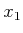and a planare known. This means that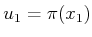, which can be substituted into (10.10) to compute the one-stage forward projection. To compute the two-stage forward projection,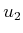is determined from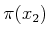for use in (10.11). A recursive formulation of the nondeterministic forward projection under a fixed plan is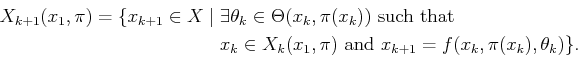(10.28)

The probabilistic forward projection in (10.10) can be adapted to use, which results in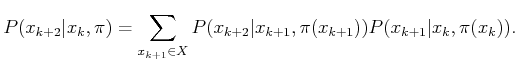(10.29)

The basic idea can be applied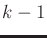times to compute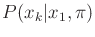.

A state transition matrix can be used once again to express the probabilistic forward projection. In (10.15), all columns correspond to the application of the action. Let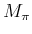, be the forward projection due to a fixed plan. Each column ofmay represent a different action because each column represents a different state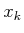. Each entry ofis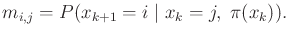(10.30)

The resultingdefines a Markov process that is induced under the application of the plan.

Steven M LaValle 2020-08-14